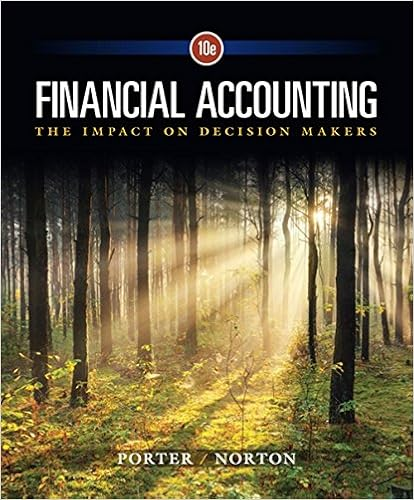# B flexible exchange rate system c small open economy

• Test Prep
• 75
• 89% (37) 33 out of 37 people found this document helpful

This preview shows page 53 - 55 out of 75 pages.

##### We have textbook solutions for you!
The document you are viewing contains questions related to this textbook.The document you are viewing contains questions related to this textbook.
Chapter 13 / Exercise 2
Financial Accounting: The Impact on Decision Makers
Norton/PorterExpert Verified
B) flexible-exchange-rate system. C) small open economy. D) large open economy. 235. In the Mundell–Fleming model, if the economy is operating at or below the natural level in the short run, then in the long run the price level will fall, the exchange rate will ______, and net exports will ______ to restore the economy to its natural rate. A) appreciate; increase B) appreciate; decrease C) depreciate; increase D) depreciate; decrease 236. If short-run equilibrium in the Mundell–Fleming model is represented by a graph with Y along the horizontal axis and the exchange rate along the vertical axis, then the IS * curve: A) slopes downward and to the right because the higher the exchange rate, the lower the level of net exports and, therefore, of short-run equilibrium income in the goods market. B) is vertical because there is only one investment level that is consistent with the world interest rate. C) is vertical because the exchange rate does not enter into the IS * equation. D) slopes downward and to the right because the higher the exchange rate, the higher the level of net exports and, therefore, of short-run equilibrium income in the goods market. 237. The Mundell–Fleming model is a ______ model for a ______ open economy. A) short-run; small B) short-run; large C) long-run; large D) long-run; small 238. According to the Mundell–Fleming model, in an economy with flexible exchange rates, expansionary fiscal policy causes the exchange rate to ______ and expansionary monetary policy causes the exchange rate to ______. A) rise; rise B) rise; fall C) fall; fall D) fall; rise Page 53
##### We have textbook solutions for you!
The document you are viewing contains questions related to this textbook.The document you are viewing contains questions related to this textbook.
Chapter 13 / Exercise 2
Financial Accounting: The Impact on Decision Makers
Norton/PorterExpert Verified
Use the following to answer question 239: Exhibit: Risk Premium 239. A small open economy with a floating exchange rate is initially in equilibrium at A with IS 1 * , LM 1 * . If the establishment of a new government in the country decreases the risk premium, then LM 1 * will shift to _____ and IS 1 * will shift to _____. A) LM 2* ; IS 2* B) LM 2* ; IS 3* C) LM 3* ; IS 2* D) LM 3* ; IS 3* 240. In a short-run model of a large open economy with a floating exchange rate, net capital outflow ______ as the domestic interest rate increases and is just equal to ______. A) decreases; the increase in net exports. B) decreases; the decrease in net exports. C) increases; the increase in net exports. D) increases; the decrease in net exports. 241. According to the Phillips curve, other things being equal, inflation depends positively on: A) expected inflation. B) the unemployment rate. C) the rate of technological change. D) the quantities of capital and labor. Page 54
•••